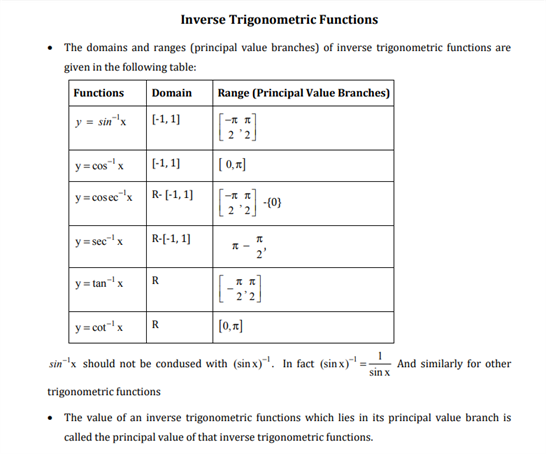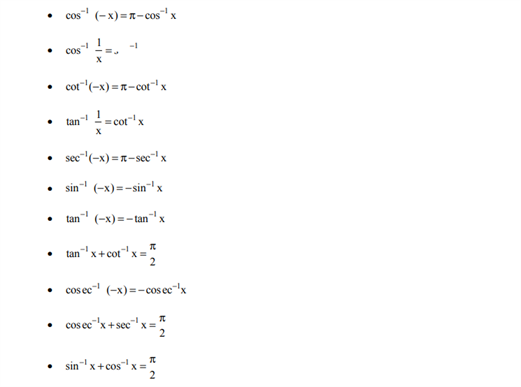# Maths Revision Notes for Class 12 Chapter 2 Inverse Trigonometric Functions.

The CBSE Class 12 Maths Revision Notes can be found here for all the chapters. Here we shall take a closer look at chapter 2 which is titled as Inverse Trigonometric Functions. In this chapter, students are introduced to the basic concepts of Trigonometric Functions and their inverses. The aim is to make a student learn and get proficient in understanding basic concepts of trigonometry such as inverse circular functions. Students who are learning these concepts will also be able to learn about other important concepts such as knowing the domain and the range of trigonometric functions.

You can find the free PDF of class 12 Maths revision notes of chapter 2 Inverse Trigonometric Functions below.You may also want to read: